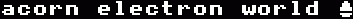### Home FAQs Library DVD

#### By Alva Parrott

Originally published in EUG #28

I wonder if anyone can help me with a small problem?

I have written a simple program for my Electron to select some random lottery numbers each week.

Although each time it is RUN, it gives a different set of numbers I find that the same sequence of 'sets' is repeated each time I switch on the computer.

I wonder if anyone can tell me how to alter the program to overcome this problem?

```       10FOR I=1 TO 8
20A=RND(49)
30PRINT "Ball number"I,A
40NEXTI
```
I asked the machine to produce eight balls to allow for duplication of numbers.

With best wishes for the future of EUG!

Alva Parrott

This kind of problem results because the computer produces the same string of numbers each time it is switched on. There is a function to randomise the random number generation, using the value of the system clock:

```      A=RND(-TIME)
```
The method used to ensure that there are not repeated numbers in your program is not very effective, because although eight numbers are selected instead of six, this does not stop the possibility of there not being six different numbers among the eight chosen. A better method is to create an array to test whether the number has been selected before. A better program is shown below:
```       10A=RND(-TIME)
20DIM n%(50)
30:
40FOR number=1 TO 6
50  REPEAT
60     A=RND(49)
70   UNTIL n%(A)=FALSE
80   n%(A)=TRUE
90   PRINT"Ball number ";number;" is ";A
100NEXT
```
What each lines does is explained below:

 10 = Randomise the numbers produced by the random number generator 20 = Set up an array variable to store which numbers have been chosen before 60 = Pick a random ball number 50/70 = If this ball has been chosen before, pick another number 80 = Store in the array that this ball has been picked 90 = Display ball number to the user 40/100 = Repeat this process for six balls

Dominic Ford, EUG #28